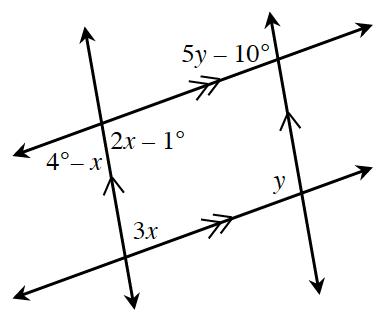### Home > CCG > Chapter Ch8 > Lesson 8.1.2 > Problem8-23

8-23.

Multiple Choice: Which equation below is not a correct statement based on the information in the diagram?

1. $3x + y = 180º$

1. $2x − 1º = 4º − x$

1. $2x − 1º = 5y − 10º$

1. $2x − 1º + 3x = 180º$

Read the Math Notes box in Lessons 2.1.1 and 2.1.4 about angle pair relationships.

B because $2x − 1º$ and $4º − x$ are a linear pair so $2x − 1º + 4° − x = 180$, not $2x − 1º = 4º − x$.